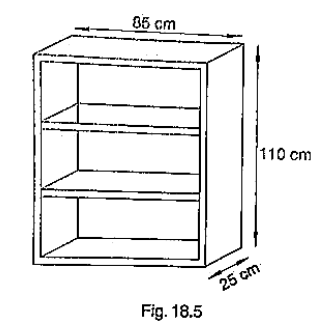# A wooden bookshelf has external dimensions as follows: Height = 110 cm, Depth = 25 cm,`
Question:

A wooden bookshelf has external dimensions as follows: Height = 110 cm, Depth = 25 cm, Breadth = 85cm (See figure 18.5). The thickness of the plank is 5cm everywhere. The external faces are to be polished and the inner faces are to be painted. If the rate of polishing is 20 paise per cm2. Find the total expenses required for polishing and painting the surface of the bookshelf.Solution:

External length of book shelf = 85 cm

Height = 110 cm

External surface area of shelf while leaving front face of shelf

lh + 2(lb + bh)

[85 * 110 + 2(85 * 25 + 25 * 110)]

$19100 \mathrm{~cm}^{2}$

Area of Front face $=\left[85^{*} 110-75^{*} 100+2\left(75^{*} 5\right)\right] \mathrm{cm}^{2}$

$=1850+750 \mathrm{~cm}^{2}$

$=2600 \mathrm{~cm}^{2}$

Area to be polished $=19100+2600 \mathrm{~cm}^{2}$

$=21700 \mathrm{~cm}^{2}$

Cost of polishing $1 \mathrm{~cm}^{2}$ area $=$ Rs. $0.20$

Cost of polishing $21700 \mathrm{~cm}^{2}$ area $=21700 * 0.20$

= Rs. 4340

Now, Length (l), breadth (b), height ( $h$ ) of each row of book shelf is $75 \mathrm{~cm}, 20 \mathrm{~cm}$ and $30 \mathrm{~cm}=\left(\frac{110-20}{3}\right)$

respectively.

Area to be painted in 1 row = 2(l + h)b + lh

$[2(75+30) * 20+75 * 30)] \mathrm{cm}^{2}$

$(4200+2250) \mathrm{cm}^{2}$

$6450 \mathrm{~cm}^{2}$

Area to be painted in 3 rows = 3 * 6450

$=$ Rs. $19350 \mathrm{~cm}^{2}$

Cost of painting $1 \mathrm{~cm}^{2}$ area $=$ Rs. $0.10$

Cost of painting $19350 \mathrm{~cm}^{2}$ area $=19350 * 0.10$

= Rs.1935

Total expense required for polishing and painting the surface of the bookshelf = 4340 + 1935

= Rs.6275